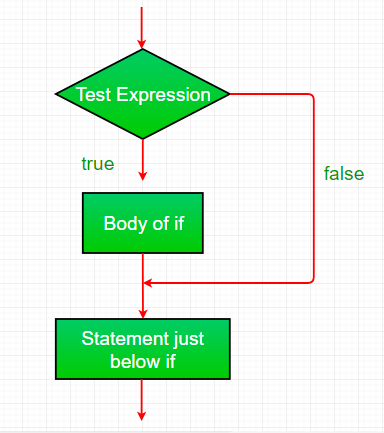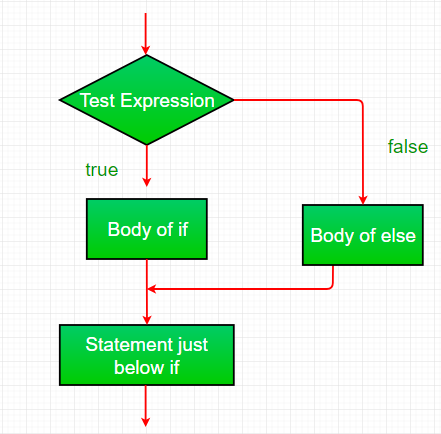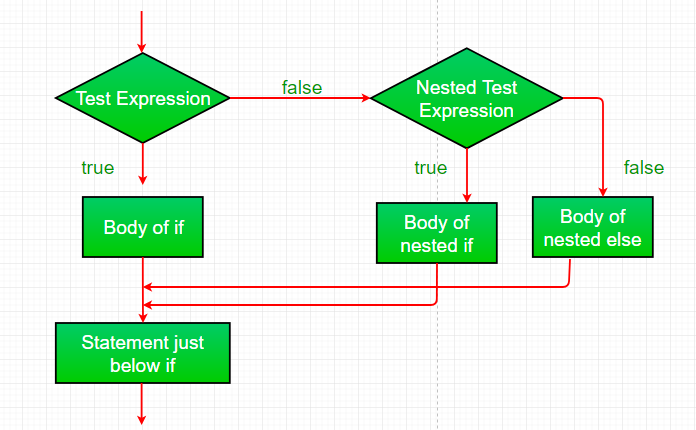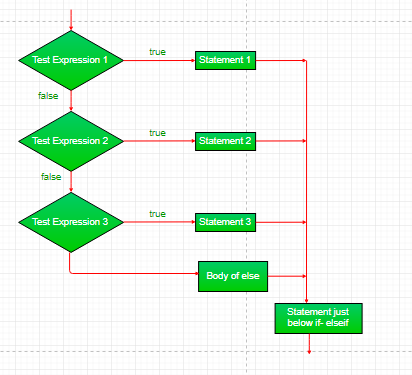# if-else Statement in JavaScript

• Difficulty Level : Basic
• Last Updated : 24 Nov, 2021

In this article, we will learn about the conditional statement (ie, if-else statement) in Javascript, along with understanding its implementation through the examples. The conditional statement will perform some action for the specific condition. If the condition meet then the particular block of action will be executed otherwise it will execute another block of action that satisfies that particular condition.

Decision Making in programming is similar to decision-making in real life. In programming also, we face some situations where we want a certain block of code to be executed when some condition is fulfilled. A programming language uses control statements to control the flow of execution of the program based on certain conditions. These are used to cause the flow of execution to advance and branch based on changes to the state of a program.

JavaScript’s conditional statements:

• if
• if-else
• nested-if

We will understand each conditional statements, its syntax, flowchart & examples. Please refer to the Switch Case in JavaScript article to understand the switch case. Let’s begin with if-statement.

if-statement: if statement is the most simple decision-making statement. It is used to decide whether a certain statement or block of statements will be executed or not i.e if a certain condition is true then a block of statement is executed otherwise not.

Syntax:

```if(condition)
{
// Statements to execute if
// condition is true
}```

Here, the condition after evaluation will be either true or false. if statement accepts boolean values – if the value is true then it will execute the block of statements under it. If we do not provide the curly braces ‘{‘ and ‘}’ after if( condition ) then by default if statement will consider the immediate one statement to be inside its block. For example,

```if(condition)
statement1;
statement2;

// Here if the condition is true, if block
// will consider only statement1 to be inside
// its block.```

Flow chart:if-condition statement

Example: This example describes the if-statement in Javascript.

## Javascript

 ``

Output:

`I am Not in if`

if-else statement: The if statement alone tells us that if a condition is true it will execute a block of statements and if the condition is false it won’t. But what if we want to do something else if the condition is false. Here comes the else statement. We can use the else statement with the if statement to execute a block of code when the condition is false.

Syntax:

```if (condition)
{
// Executes this block if
// condition is true
}
else
{
// Executes this block if
// condition is false
}```

Flow chart:if-else statement

Example: This example describes the if-else statement in Javascript.

## JavaScript

 ``

Output:

`i is smaller than 15`

nested-if statement: A nested if is an if statement that is the target of another if or else. Nested if statements mean if statement inside another if statement. Yes, JavaScript allows us to nest if statements within if statements. i.e, we can place an if statement inside another if statement.

Syntax:

```if (condition1)
{
// Executes when condition1 is true
if (condition2)
{
// Executes when condition2 is true
}
}```

Flow chart:nested-if statement

Example: This example describes the nested-if statement in Javascript.

## JavaScript

 ``

Output:

```i is smaller than 15
i is smaller than 12 too```

if-else-if ladder statement: Here, a user can decide among multiple options.The if statements are executed from the top down. As soon as one of the conditions controlling the if is true, the statement associated with that if is executed, and the rest of the ladder is bypassed. If none of the conditions is true, then the final else statement will be executed.

Syntax:

```if (condition)
statement;
else if (condition)
statement;
.
.
else
statement;```

Flow chart:Example: This example describes the if-else-if ladder statement in Javascript.

## JavaScript

 ``

Output:

`i is 20`

Supported Browsers: